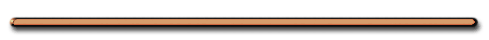Conservation of Momentum   Ex) 12,600 kg railroad car travels on a frictionless surface with a constant speed of 18.0 m/s. A 5350 kg load at rest is dropped onto the car, what will the car's new speed be?   Total momentum before = total momentum after m1v1 = m2v2           V2 = 12.6 m/s   Ex) An atomic nucleus moving at 420 m/s emits an alpha particle in the direction of its motion and the remaining nucleus slows to 350 m/s. If the alpha particle has a mass of 4.0 µ and the original nucleus has a mass of the 222 µ, what speed does the original alpha particle have when it is emitted?     No need to convert mass to kg. Why?   Before Original nucleus = mass = 222 µ, v =  420 m/s After alpha particle  = mass of 4.0 µ v = ? New Nucleus = 218 µ  (alpha lost) v = 350 m/s Speed of original alpha particle have when it is emitted?   mom nucleus = mom alpha + mom new nucleus mv = mava + mnvn     v = 4.2 x 103 m/s©Tony Mangiacapre., -  All Rights Reserved [Home] Established 1995 Use any material on this site (w/ attribution)